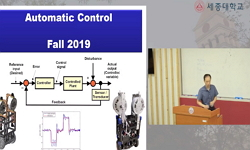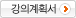### 주메뉴

### 자동제어

• 세종대학교
• 곽관웅• 주제분류
공학 >기계ㆍ금속 >기계공학
• 강의학기
2019년 2학기
• 조회수
43,316
• 평점
4.6/5.0 (5)
강의계획서Chapter 1.Introduction to Feedback Control (1)#### 차시별 강의1.Chapter 1.Introduction to Feedback Control (1)Introduction to Feedback Control (2)The Laplace Transform2.The Laplace Transform (Inverse Laplace Transform, Partial Fraction Expansion)Solving linear time-invariant differential equations using L.T.Chapter 2. Modeling of Dynamic systemsModels of Electric CircuitsModels of Electromechanical SystemsDC motor (1)DC motor (2)MATLAB/Simulink Basics (1)3.MATLAB/Simulink Basics (2)Chapter 3. Dynamic Response4.Block DiagramsBlock Diagram reduction using MATLAB5.Signal Flow GraphsMasons RuleLinear System Analysis using MATLAB/SimulinkEffect of Pole Locations6.Time-Domain SpecificationsEffect of Zeros and Additional Poles2nd order system, higher order systems, Stability analysisStability, Stability of LTI Systems7.Stability(Routh is Stability CriterionRough-hurwitz Criterion: Special Cases) - (1)Stability(Routh is Stability CriterionRough-hurwitz Criterion: Special Cases) - (2)Relative Stability8.A First Analysis of Feedbackstatic error constant, steady-state error for nonunity feedbackSteady State Error and PID controller9.Root Locus TechniquesRoot-Locus Analysis (Root-Locus plots, pole-zero cancellation)10.Root-Locus Analysis (Nonminimum phase system, positive feedback system, conditionally stable system)Control Systems Design by the Root-Locus Method (Lead compensation)Control Systems Design by the Root-Locus Method (Lag compensation, Lead-Lag compensation)11.Control Systems Design by the Root-Locus Method (Lead-Lag compensation, paralle compensation, velocity feedback)Frequency Response Analysis (Bode plots of first-order factors) Frequency Response Analysis (Bode plots of first-order & quadratic factors)12.Frequency Response Analysis (system types and log-magnitude curve)Frequency Response Analysis (polar plots)Frequency Response Analysis (Nyquist stability criterion)Frequency Response Analysis (Nyquist stability criterion, relative stability)Frequency Response Analysis (Nyquist diagram , Gain Margin and Phase Margin via the Nyquist Diagram, Gain Margin and Phase Margin via the Bode Plot)#### 연관 자료#### 사용자 의견

강의 평가를 위해서는 로그인 해주세요.
whqjatlr159 2022-08-28 22:05
강의력은 정말 좋으신데 Simulink 사용하실 때, 컴퓨터 화면 적용이 안되서 어떤거 하고계시는지 이해하기가 너무 힘들어요 ㅠㅠ
운영자2021-10-05 10:33
KOCW입니다. 문의내용을 세종대학교로 전달했습니다. 답변이 오는 대로 안내드리도록 하겠습니다.
yyas88 2021-10-04 22:59
진짜 너무 이해가 잘되고 공부할때 도움이 많이됩니다.ㅠㅠㅠㅠㅠ혹시 이후 강의는 없을까요..?
운영자2020-09-28 09:17
KOCW입니다. 세종대학교에서는 저작권등의 사유로 KOCW에 강의자료를 제공하지 않습니다. 양해 부탁드립니다.
juhyejeong 2020-09-26 19:37
안녕하세요. 혹시 강의자료를 받을 수 있을까요?

#### 이용방법

• 동영상 유형 강의 이용시 필요한 프로그램 [바로가기]

※ 강의별로 교수님의 사정에 따라 전체 차시 중 일부 차시만 공개되는 경우가 있으니 양해 부탁드립니다.